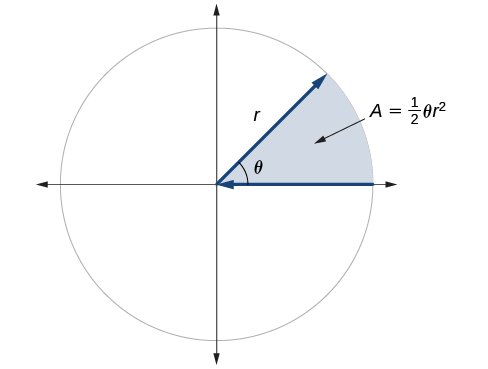# 7.1 Angles  (Page 7/29)

 Page 7 / 29

Find the arc length along a circle of radius 10 units subtended by an angle of $\text{\hspace{0.17em}}215°.$

## Finding the area of a sector of a circle

In addition to arc length, we can also use angles to find the area of a sector of a circle . A sector is a region of a circle bounded by two radii and the intercepted arc, like a slice of pizza or pie. Recall that the area of a circle with radius $\text{\hspace{0.17em}}r\text{\hspace{0.17em}}$ can be found using the formula $\text{\hspace{0.17em}}A=\pi {r}^{2}.\text{\hspace{0.17em}}$ If the two radii form an angle of $\text{\hspace{0.17em}}\theta ,$ measured in radians, then $\text{\hspace{0.17em}}\frac{\theta }{2\pi }\text{\hspace{0.17em}}$ is the ratio of the angle measure to the measure of a full rotation and is also, therefore, the ratio of the area of the sector to the area of the circle. Thus, the area of a sector is the fraction $\text{\hspace{0.17em}}\frac{\theta }{2\pi }\text{\hspace{0.17em}}$ multiplied by the entire area. (Always remember that this formula only applies if $\text{\hspace{0.17em}}\theta \text{\hspace{0.17em}}$ is in radians.)

## Area of a sector

The area of a sector    of a circle with radius $\text{\hspace{0.17em}}r\text{\hspace{0.17em}}$ subtended by an angle $\text{\hspace{0.17em}}\theta ,$ measured in radians, is

$A=\frac{1}{2}\theta {r}^{2}$The area of the sector equals half the square of the radius times the central angle measured in radians.

Given a circle of radius $\text{\hspace{0.17em}}r,$ find the area of a sector defined by a given angle $\text{\hspace{0.17em}}\theta .$

1. If necessary, convert $\text{\hspace{0.17em}}\theta \text{\hspace{0.17em}}$ to radians.
2. Multiply half the radian measure of $\text{\hspace{0.17em}}\theta \text{\hspace{0.17em}}$ by the square of the radius $\text{\hspace{0.17em}}r:\text{​}A=\frac{1}{2}\theta {r}^{2}.$

## Finding the area of a sector

An automatic lawn sprinkler sprays a distance of 20 feet while rotating 30 degrees, as shown in [link] . What is the area of the sector of grass the sprinkler waters?The sprinkler sprays 20 ft within an arc of   30° .

First, we need to convert the angle measure into radians. Because 30 degrees is one of our special angles, we already know the equivalent radian measure, but we can also convert:

The area of the sector is then

$\begin{array}{ccc}\hfill \text{Area}& =& \frac{1}{2}\left(\frac{\pi }{6}\right){\left(20\right)}^{2}\hfill \\ & \approx & 104.72\hfill \end{array}$

So the area is about

In central pivot irrigation, a large irrigation pipe on wheels rotates around a center point. A farmer has a central pivot system with a radius of 400 meters. If water restrictions only allow her to water 150 thousand square meters a day, what angle should she set the system to cover? Write the answer in radian measure to two decimal places.

1.88

## Use linear and angular speed to describe motion on a circular path

In addition to finding the area of a sector, we can use angles to describe the speed of a moving object. An object traveling in a circular path has two types of speed. Linear speed is speed along a straight path and can be determined by the distance it moves along (its displacement ) in a given time interval. For instance, if a wheel with radius 5 inches rotates once a second, a point on the edge of the wheel moves a distance equal to the circumference, or $\text{\hspace{0.17em}}10\pi \text{\hspace{0.17em}}$ inches, every second. So the linear speed of the point is $\text{\hspace{0.17em}}10\pi \text{\hspace{0.17em}}$ in./s. The equation for linear speed is as follows where $\text{\hspace{0.17em}}v\text{\hspace{0.17em}}$ is linear speed, $\text{\hspace{0.17em}}s\text{\hspace{0.17em}}$ is displacement, and $\text{\hspace{0.17em}}t\text{\hspace{0.17em}}$ is time.

$v=\frac{s}{t}$

Angular speed results from circular motion and can be determined by the angle through which a point rotates in a given time interval. In other words, angular speed    is angular rotation per unit time. So, for instance, if a gear makes a full rotation every 4 seconds, we can calculate its angular speed as 90 degrees per second. Angular speed can be given in radians per second, rotations per minute, or degrees per hour for example. The equation for angular speed is as follows, where $\text{\hspace{0.17em}}\omega \text{\hspace{0.17em}}$ (read as omega) is angular speed, $\text{\hspace{0.17em}}\theta \text{\hspace{0.17em}}$ is the angle traversed, and $\text{\hspace{0.17em}}t\text{\hspace{0.17em}}$ is time.

#### Questions & Answers

write down the polynomial function with root 1/3,2,-3 with solution
if A and B are subspaces of V prove that (A+B)/B=A/(A-B)
write down the value of each of the following in surd form a)cos(-65°) b)sin(-180°)c)tan(225°)d)tan(135°)
Prove that (sinA/1-cosA - 1-cosA/sinA) (cosA/1-sinA - 1-sinA/cosA) = 4
what is the answer to dividing negative index
In a triangle ABC prove that. (b+c)cosA+(c+a)cosB+(a+b)cisC=a+b+c.
give me the waec 2019 questions
the polar co-ordinate of the point (-1, -1)
prove the identites sin x ( 1+ tan x )+ cos x ( 1+ cot x )= sec x + cosec x
tanh`(x-iy) =A+iB, find A and B
B=Ai-itan(hx-hiy)
Rukmini
what is the addition of 101011 with 101010
If those numbers are binary, it's 1010101. If they are base 10, it's 202021.
Jack
extra power 4 minus 5 x cube + 7 x square minus 5 x + 1 equal to zero
the gradient function of a curve is 2x+4 and the curve passes through point (1,4) find the equation of the curve
1+cos²A/cos²A=2cosec²A-1
test for convergence the series 1+x/2+2!/9x3ByByByByBy